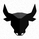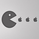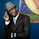2194 views
This Oscillator helps to identify the ending of waves, good to get entries and exits. Now, with a tolerance line.
```study("Snake Oscillator","SNK_OSC")

len = input(25,"Length")

h = ema(high,len)
l = ema(low,len)

hp = h/h[len]
lp = l/l[len]

avg = avg(hp,lp)

havg = ema(highest(avg,len),len)
lavg = ema(lowest(avg,len),len)

avg2 = avg(havg,lavg)

dif = havg - avg2

pa = plot(avg,color=gray)
ph = plot(havg,color=gray)
pl = plot(lavg,color=gray)

plot(havg+dif,color=black)
plot(lavg-dif,color=black)

fill(ph,pa,color=red,transp=60)
fill(pl,pa,color=green,transp=60)
```hp = h/h // This provides the porcentage of change of ema(high, len) considered len periods back
lp = l/l // same of hp, but with lows

avg = avg(hp,lp) // Average of porcentage of change of low and High

havg = ema(highest(avg,len),len) // Highest avg of change of len periods back amortized by ema
lavg = ema(lowest(avg,len),len) //Lowest avg of change of len periods back amortized by ema

--

Idea is check if the trend is making the same proportion of highs and lows in a trend move.